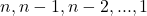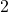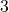# Programming Concepts: Tail Recursion

Tail recursion returns the result of a call to the function itself, potentially with modified parameters, and absolutely not mess with the return value with anything dependent on the caller’s state, such as local variables. This means absolutely no operations involving caller variable between the recursive call and the return value. The recursive call must be at the tail (end branch) of the caller. Here’s a GCD (Euler’s algorithm) example:

int gcd(a, b)
{
if(b=0) {return a;}
return gcd(b, a%b);
}

One way to visualize this is that if you put the recursive call at the very end as return value, what you are effectively doing is not really recursion, but looping the same code (calling itself by substituting a parameter with the return value) until you hit a break condition and the innermost call (or the last repeat of the function body) contains your answer.

For this example, I intentionally do this in a generic template way which is not tailored to your program context

int gcd_iter(int a, int b)
{
// This is what gcd(a=b, b=a%b) till b=0 does
while(true) {
if(b==0) {return a;}

// This emulates pass by value
// in the arguments of the function call
int a1 = b;
int b1 = a % b;

a = a1;
b = b1;
}
}

Only one temporary (‘local’ variable in the recursive call) a1 or b1 is needed so they won’t overwrite each other (it’d be the same chicken and egg problem in variable swap) and the other could be reused, but this is up to the compiler to optimize away as this example is to show a template where the tail recursion can be unconditionally converted into loops.

Tail recursion means your recursive algorithm simply march all the way down (forward) and you are done at the innermost call, where the stack unwinding in a tail recursion is purely a chore for relaying the (terminated) answer back to the top of the call which you wouldn’t have needed to do so had you been using a loop since the answer stays within the same variable scope.

Conceptually, the recursive call marches down like this:

int a1, b1;
int gcd_unroll(int a, int b)
{
if(b==0) {return a;}
// Call 1: gcd(b, a%b)
{
// pass by val
a1=b; b1=a%b;
a=a1; b=b1;
// body
if(b==0) {return a;}
// Call 2:
{
// pass by val
a1=b; b1=a%b;
a=a1; b=b1;
// body
if(b==0) {return a;}
// Call 3:
{
// pass by val
a1=b; b1=a%b;
a=a1; b=b1;
// body
if(b==0) {return a;}
}
}
}
}

As long as the closing braces ‘}’ are contiguous (i.e. no additional work after the recursive calls completed), the code marches in on-direction, namely forward (down) only and there’s no need to remember the earlier values from the ‘caller’ as the braces closes.

Non-tail recursion however, relies on the caller to ‘add its own insight (use caller’s local variables)’ relying on the half-answer (value) returned by the inner call as soon as the innermost call ends and propagates up.

So the code marches down (forward), hit a break, and meaningfully (i.e. there’s something to be done in the process) marches back up (in reverse order), so it’s not equivalent to a loop which marches only forward. For example, this factorial program do not tail-recurse and therefore not directly translatable to a loop without adding extra storage:

int factorial_stack(int n) {
if(n==1) {return 1;}
return n*factorial_stack(n-1);
}

If it’s just something simple like multiplying or adding constants after the recursive call, a very smart compiler still stands a chance to refactor your code by introducing a counter on how many levels the calls went down and repeat the simple constant operation after the return call that many times. Relying on compiler like this is risky business, so make sure you check the assembly or benchmark to see if the compiler is indeed this smart to not start new stack for it.

However, if you start using (usually local) variables within the caller’s state (stack) to process the return value (namely ‘n’ in the example above), you are implicitly using the call stack as your stack data structure to temporarily hold these variables which you will later use (specifically when the stack unwinds) to process the value returned from the recursive call, so the stack has to grow because what you’re doing translates to pushing the variable needed for post-processing the return value from the recursive call onto a stack as you march down, and popping them (and use them) on your way up (return/unwinding). Here’s the conceptual equivalent:

int factorial_stack(int n)
{
if(n==1) {return 1;}
// Call 1: factorial_stack(n-1)
{
// pass by val
int n1=n-1; n=n1;
// body
if(n==1) {return 1;}
// Call 2:
{
// pass by val
int n2=n-1; n=n2;
// body
if(n==1) {return 1;}
// Call 3:
{
// pass by val
int n3=n-1; n=n3;
// body
if(n==1) {return 1;}

{
...
}
return n3*1;
}
return n2*(n3*1);
}
return n1*(n2*(n3*1));
}
return n*(n1*(n2*(n3*1)));
}

Note that the n1, n2, n3, … cannot be recycled. A different value is stored on the stack as the recursion goes deeper. What you are really doing is pushingonto a stack data structure (could be external heap if it’s not call stack), and after you hit, you pop the items back from the stack in reverse order to accumulate a product starting from 1 (innermost call) and upward, therefore doing

((((1*2)*3)*4)* ... (N-1))*N

which is therefore from left-to-right

1*2*3*...*(N-1)*N

instead of what iteration intuitively does from left-to-right if we count down:

N*(N-1)*(N-2)*....*2*1

because the parenthesis (function composition) starts by multiplyingwithfirst, thenN*((N-1)*((N-2)*....*(3*(2*1))))

in the same order as the non-tail recursion, which starts by multiplyingwithfirst, then((((1*2)*3)*4)* ... (N-1))*N

In the GCD example, tail recursion’s actions solely happens before the recursive call, so the operations are in the order of the function call. However in the factorial_stack() example, non-tail recursion’s action solely happens after the recursive call, so all the core actions are in the order which the stack unwinds, which is the opposite order of function calls.

Tree transversal can also be visualized the same way. Pre-order transversal is a tail recursion. The action happens in the order the function calls.

Using the function call stack to replace a dedicated external stack data structure (often on the heap though you can allocate a dedicated fixed-size-limited stack at the top level caller) also means you’re lugging the baggage of other local variables that are no longer needed just for that one or few local variables (for processing return values after the recursive call) that you wanted an implicit stack for.

So judge for yourself how much space overhead wastage on the stack you’d sacrifice to avoid a dedicated stack if you cannot restructure your program (often by introducing an extra storage variable/parameter) to make tail recursion or loops possible.

In the factorial example above, it can be made tail recursive:

int factorial_tail(int n, int prod_accumulated)
{
// Seed prod (accumulator) with 1
if(n==1) {return prod_accumulated;}
return factorial_tail(n-1, prod_accumulated*n);
}

The trick is to start accumulating the product before the recursive call (i.e. at the caller), not after. This means you need to provide extra storage to start the accumulation at the top (outermost) call, not deep down in the recursion (innermost). In this example, the order of accumulation from left to right is

1_{\mathrm{seed}}*N*(N-1)*...*2*1

while the non-tail recursion’s product accumulation order from left to right is

1*2*3*...*N-1*N

The tail-recursive factorial_tail() above directly translates the same intuitive code a middle school kid can write:

int factorial_loop(int n)
{
int prod=1;
while(n>1) {
prod=n*prod;
--n;
}
return prod;
}

This is the code that illustrates the order of execution of the head recursion factorial_stack() with printouts,

int factorial_stack(int n) {
if(n==1) {return 1;}
int prod = n*factorial_stack(n-1);
cout << "factorial_stack prod: " << prod << endl;
return prod;
}

/* Output:
factorial_stack prod: 2
factorial_stack prod: 6
factorial_stack prod: 24
factorial_stack prod: 120
*/

factorial_reverse_loop() shows the order of execution intention (not the actual mechanics as a stack is not involved) of the factorial_stack() that shows what the recursion in general is travelling downwards then back up, which tail recursion is the ability to short circuit the backing up part.

int factorial_reverse_loop(int N)
{
int n=N;
while (n>1) {
cout << "Emulate head recursion n: " << n << endl;
--n;
}
cout << "n after the down loop: " << n <<endl;

int prod_accum=1;
while (n<=N) {
prod_accum = prod_accum*n;
cout << "Multiplied by " << n << " , Product is " << prod_accum << endl;
n++;
}
return prod_accum;
}

/* Output
n after the down loop: 1
Multiplied by 1 , Product is 1
Multiplied by 2 , Product is 2
Multiplied by 3 , Product is 6
Multiplied by 4 , Product is 24
Multiplied by 5 , Product is 120
*/

The STL stack equivalent of the factorial_stack() in loop form is:

int factorial_explicit_stack(const int N) {
int n = N;

stack<int> s;
while (n>1) {
s.push(n);
cout << "Item pushed on stack: " << n << endl;
--n;
}
// n will be 1 at this point
// There'd be N-1 items on stack
// as 1 doesn't need to be stored

// Compensate for the unnecessary drop to 1 at the end
n++;

int prod_accum=1;
while (n<=N) {
prod_accum = prod_accum*s.top();
cout << "Item read on stack: " << s.top() << " while n=" << n << endl;
s.pop();
n++;
}
return prod_accum;
}

/* Output:
Item pushed on stack: 5
Item pushed on stack: 4
Item pushed on stack: 3
Item pushed on stack: 2
Item read on stack: 2 while n=2
Item read on stack: 3 while n=3
Item read on stack: 4 while n=4
Item read on stack: 5 while n=5
120
*/

Of course the stack is not necessary for computing factorial, but this shows the implicit stack that’s hidden in a non-tail recursion.